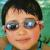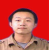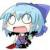# VS2013中的C++11新特性已翻译 100%

## 介绍

Visual C++ 2013 Preview 在6月发布了,C++开发者又找到一个编译器可以更好的支持ISO C++ 11 的特性了.本文介绍了这些新的特性并附有代码实例.

### 原始字符串字面值

VC++ 2013现在支持原始字符串字面值了。注意：它并不支持统一码字符串字面值。一个原始字符串字面值允许你避免转义那些在HTML，XML和正则表达式里运用得得心应手的特殊字符。下面是一个示例用法：

`auto s1 = R"(This is a "raw" string)";`

`auto s2 = R"QQ(Example: R"(This is my raw string)")QQ";`

```auto s3 = R"(<tr>
<td>data</td>
</tr>)";```

## 可变参数模板

```// Variadic template declaration
template<typename... Args> class Test;

// Specialization 1
template<typename T> class Test<T>
{
public:
T Data;
};

// Specialization 2
template<typename T1, typename T2> class Test<T1, T2>
{
public:
T1 Left;
T2 Right;
};

void Foo()
{
Test<int> data;
data.Data = 24;

Test<int, int> twovalues;
twovalues.Left = 12;
twovalues.Right = 15;
}```

```template<typename... Args> class Test
{
public:
size_t GetTCount()
{
return sizeof...(Args);
}
};

// . . .

Test<int> data;
size_t args = data.GetTCount(); //1

Test<int, int, char*> data2;
args = data2.GetTCount(); //3

Test<int, float> data3;
args = data3.GetTCount(); //2```

```template<typename... Args> class Test;

// Specialization for 0 arguments
template<> class Test<>
{
};

// Specialization for at least 1 argument

template<typename T1, typename... TRest> class Test<T1, TRest...>
: public Test<TRest...>
{
public:
T1 Data;

// This will return the base type
Test<TRest...>& Rest()
{
return *this;
}
};

void Foo()
{
Test<int> data;
data.Data = 24;

Test<int, int> twovalues;
twovalues.Data = 10;
// Rest() returns Test<int>
twovalues.Rest().Data = 11;

Test<int, int, char*> threevalues;
threevalues.Data = 1;
// Rest() returns Test<int, int>
threevalues.Rest().Data = 2;
// Rest().Rest() returns Test<char*>
threevalues.Rest().Rest().Data = "test data";
}```

### Tuple的实现

```// tuple
template<class... _Types> class tuple;

// 空tuple
template<> class tuple<> {};

// 递归的tuple定义
template<class _This,
class... _Rest>
class tuple<_This, _Rest...>
: private tuple<_Rest...>
{
public:
_This _Myfirst;
};```

```// tuple_element
template<size_t _Index, class _Tuple> struct tuple_element;

// select first element
template<class _This, class... _Rest>
struct tuple_element<0, tuple<_This, _Rest...>>
{
typedef _This& type;
typedef tuple<_This, _Rest...> _Ttype;
};

// recursive tuple_element definition
template <size_t _Index, class _This, class... _Rest>
struct tuple_element<_Index, tuple<_This, _Rest...>>
: public tuple_element<_Index - 1, tuple<_Rest...> >
{
};```

```// get reference to _Index element of tuple
template<size_t _Index, class... _Types> inline
typename tuple_element<_Index, tuple<_Types...>>::type
get(tuple<_Types...>& _Tuple)
{
typedef typename tuple_element<_Index, tuple<_Types...>>::_Ttype _Ttype;
return (((_Ttype&) _Tuple)._Myfirst);
}```

```tuple<int, char> t1;
get<0>(t1) = 959;
get<1>(t1) = 'A';

auto v1 = get<0>(t1);
auto v2 = get<1>(t1);```

### 代理构造函数

```class Error
{
public:
Error()
{
Init(0, "Success");
}

Error(const char* message)
{
Init(-1, message);
}

Error(int errorCode, const char* message)
{
Init(errorCode, message);
}

private:
void Init(int errorCode, const char* message)
{
//...
}
};```

```class Error
{
public:
Error() : Error(0, "Success")
{
}

Error(const char* message) : Error(-1, message)
{
}

Error(int errorCode, const char* message)
{
// ...
}
};```

## 函数模板中的默认模板参数

```template <typename T = int> void Foo(T t = 0) { }

// error C4519: default template arguments are only
// allowed on a class template```
Visual C++ 2013 能够顺利编译这些代码，模板参数推断也能正确进行。
```Foo(12L); // Foo<long>
Foo(12.1); // Foo<double>
Foo('A'); // Foo<char>
Foo(); // Foo<int>```

```template <typename T> class Manager
{
public:
void Process(T t) { }
};

template <typename T> class AltManager
{
public:
void Process(T t) { }
};

template <typename T, typename M = Manager<T>> void Manage(T t)
{
M m;
m.Process(t);
}

Manage(25); // Manage<int, Manager<int>>
Manage<int, AltManager<int>>(25); // Manage<int, AltManager<int>>```

```template <typename B, typename T = int> void Bar(B b = 0, T t = 0) { }

Bar(10); // Bar<int, int>
Bar(10L); // Bar<long, int>
Bar(10L, 20L); // Bar<long, long>
Bar(); // will not compile```

```template <typename T = int> void Foo(T t = 0) { }
template <typename B, typename T = int> void Foo(B b = 0, T t = 0) { }

Foo(12L); // will not compile
Foo(12.1); // will not compile
Foo('A'); // will not compile
Foo(); // Foo<int>```

## 显式转换运算符

```class Test1
{
public:
explicit Test1(int) { }
};

void Foo()
{
Test1 t1(20);
Test1 t2 = 20; // will not compile
}```

```class Test1
{
public:
explicit Test1(int) { }
};

class Test2
{
int x;
public:
Test2(int i) : x(i) { }
operator Test1() { return Test1(x); }
};

void Foo()
{
Test2 t1 = 20;
Test1 t2 = t1; // will compile
}```

```class Test2
{
int x;
public:
Test2(int i) : x(i) { }
explicit operator Test1() { return Test1(x); }
};

void Foo()
{
Test2 t1 = 20;
Test1 t2 = (Test1)t1; // this compiles
Test1 t3 = t1; // will not compile
}```

```class Test3
{
public:
operator bool() { return true; }
};

void Foo()
{
Test3 t3;
if (t3)
{
}

bool b = t3;
}```

```class Test3
{
public:
explicit operator bool() { return true; }
};

void Foo()
{
Test3 t3;
if (t3) // this compiles!
{
}

bool b = t3; // will not compile
}```

### 评论(42)2013看上去不错，准备买个256G SSD，重装系统再升级visual studio的支持C++11 没有g++做得好啊,见链接:
http://cpprocks.com/c11-compiler-support-shootout-visual-studio-gcc-clang-intel/

x

#### 引用来自“有人知是燕归来”的评论#### 引用来自“有人知是燕归来”的评论#### 引用来自“有人知是燕归来”的评论#### 引用来自“有人知是燕归来”的评论